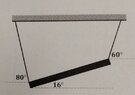# Tension and Torque Problem

• marinarasauce
In summary, the conversation discusses a problem where the person is unsure of how to start. They are advised to draw a Free Body Diagram (FBD) and write equations to sum all of the forces and torques to zero. It is also mentioned that an axis needs to be chosen for the torque equation.

#### marinarasauce

Homework Statement
An 87 cm long metal bar has a mass of .695 kg and is supported by 2 strings

-Find the tension in each string

-Find the center of the mass for the bar relative to the left end of the bar
Relevant Equations
I think we use
Torque= F r sin theta....?
I have no idea where to start or how to do this. I haven't learned this in lecture yet

#### Attachments

•IMG_20221101_130041495~2.jpg
38.5 KB · Views: 51
Welcome to PF.

marinarasauce said:
I have no idea where to start or how to do this.
With problems like this it's best to start with a Free Body Diagram (FBD) of the object that shows all of the forces acting on it (in this case the two strings pulling up at their contact points with the angles, along with the force of gravity acting down on the bar at it's center of gravity point). Once you have your FBD drawn and labeled, you write the equations to sum all of the forces to zero and to sum all of the torques to zero.

Try sketching that FBD for us and upload it using the "Attach files" link below the Edit window.

EDIT -- Beat out by @kuruman again!To elaborate on @berkeman 's reply, you need to pick an axis for the torque equation. Generally one chooses a point at which some of the forces act so that those do not feature in the equation. Occasionally it pays to pick two axes and write the torque equation for each, but for a single free body that is usually redundant since it can be deduced from the other torque equation and the linear equations.